# Calculating pressure Aseel Samaro Introduction Calculating pressure is

• Slides: 15
Download presentation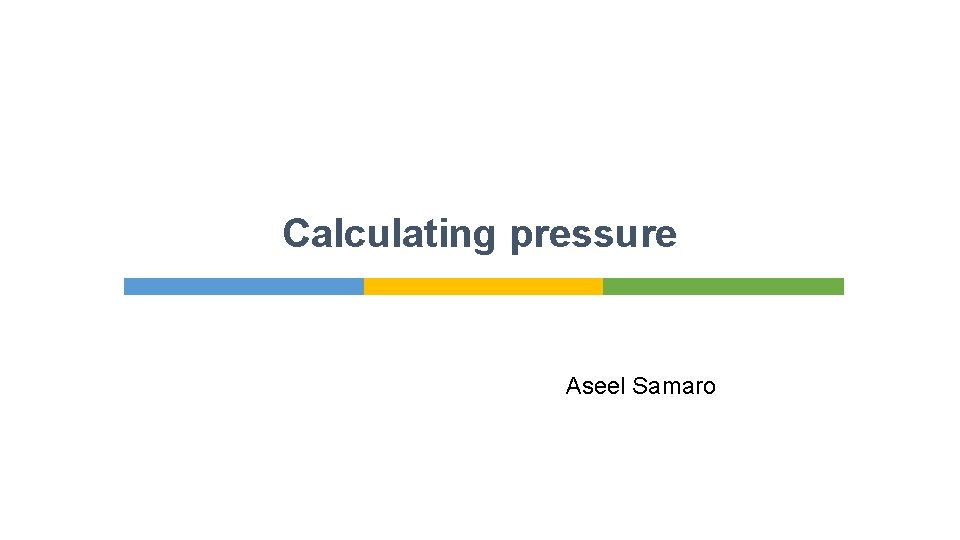Calculating pressure Aseel SamaroIntroduction § Calculating pressure is important to engineers. § For example, the foundations of a building have to carry the full downwards force of its weight. § There have been cases of buildings collapsing when the pressure on the foundations was too large. § Engineers calculate the area needed for the foundations to support the structure.Calculating pressure § Pressure tells us how much force is applied over an area. The formula used to calculate pressure is: § Force is measured in newtons (N) and area is measured in square metres, so the pressure is measured in newtons per square metre (N/m 2). § The unit for pressure is the pascal (Pa). 1 Pa is exactly the same as 1 N/m 2.What is the unit for pressure? What is the formula for calculating pressure? If we calculated pressure using force in newtons and area in square centimetres, what unit would the answer be in?What is the unit for pressure? pascal (Pa); N/m 2 What is the formula for calculating pressure? P = F A If we calculated pressure using force in newtons and area in square centimetres, what unit would the answer be in? N/cm 2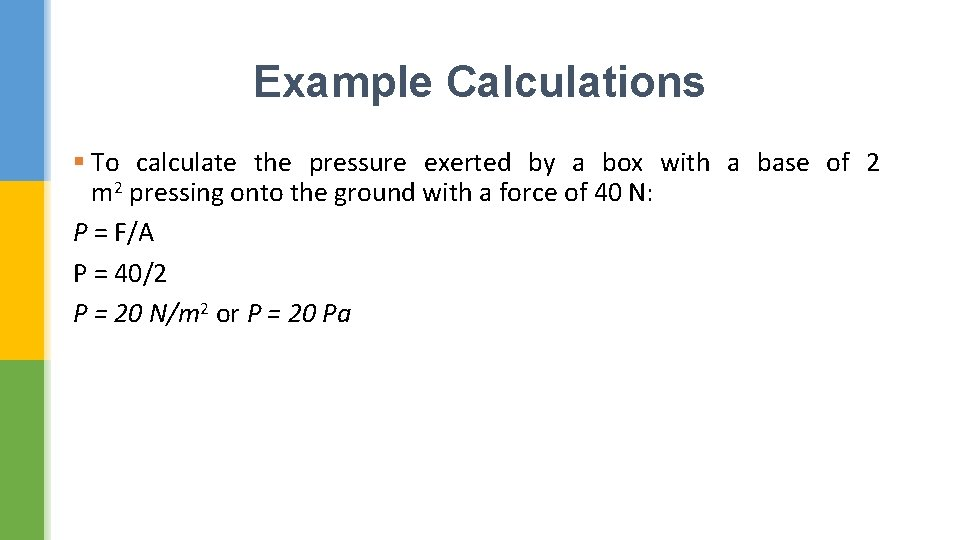Example Calculations § To calculate the pressure exerted by a box with a base of 2 m 2 pressing onto the ground with a force of 40 N: P = F/A P = 40/2 P = 20 N/m 2 or P = 20 Pa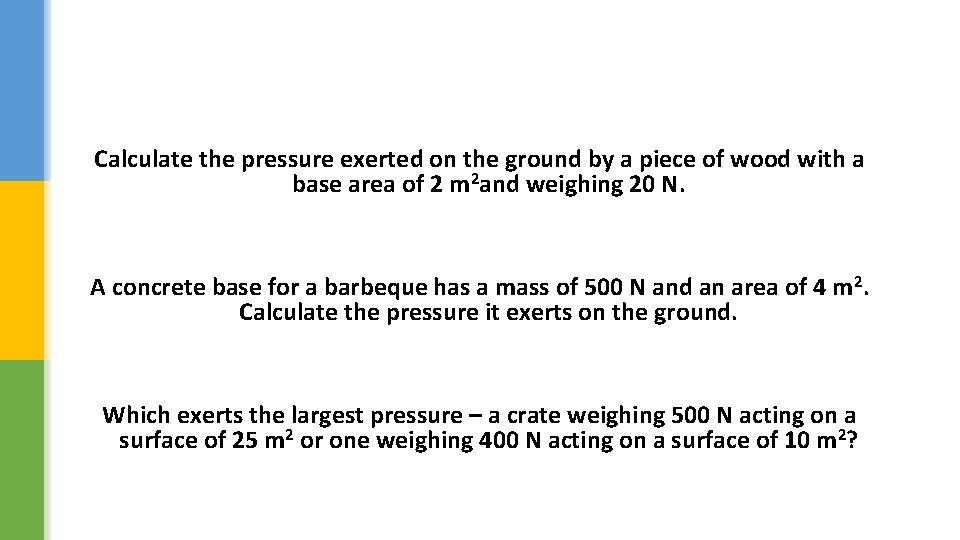Calculate the pressure exerted on the ground by a piece of wood with a base area of 2 m 2 and weighing 20 N. A concrete base for a barbeque has a mass of 500 N and an area of 4 m 2. Calculate the pressure it exerts on the ground. Which exerts the largest pressure – a crate weighing 500 N acting on a surface of 25 m 2 or one weighing 400 N acting on a surface of 10 m 2?Calculate the pressure exerted on the ground by a piece of wood with a base area of 2 m 2 and weighing 20 N. 10 Pa A concrete base for a barbeque has a mass of 500 N and an area of 4 m 2. Calculate the pressure it exerts on the ground. 125 Pa Which exerts the largest pressure – a crate weighing 500 N acting on a surface of 25 m 2 or one weighing 400 N acting on a surface of 10 m 2? pressures are 20 Pa and 40 Pa respectively; the second crate exerts the greater pressureSolving pressure problems § If you know what the values of two of the quantities in the pressure formula are, you can calculate third quantity by rearranging the formula. § P = F/A or F=Px. A § An engineer can work out the strength and size of designs needed to withstand certain pressures. For example, a bridge needs to take loads up to 45 000 N. § It is built on ground that can withstand 5000 Pa. To calculate the area needed for the bridge supports: § A=F/P = 45000/5000 = 9 m 2 § Care must be taken to use the correct units for all quantities. The area is in square metres because the force was in newtons and the pressure was in pascalsHow could you work out the pressure that this piano exerts on the floor?An engineer wants the floor of a car to be able to take a total force of 12 000 N from all the seats. The floor can take a pressure of 24 000 Pa. What area must the seat supports be? When someone is on a trampoline they may be sitting down or on their feet. üExplain whether the pressure on the trampoline is higher when sitting or standing. üHow could you calculate the difference in the pressures exerted when sitting or standing?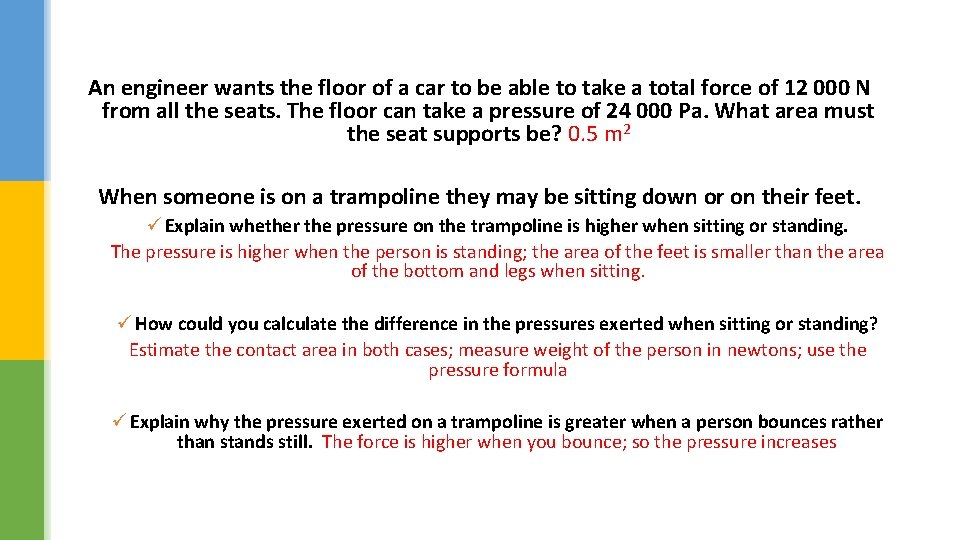An engineer wants the floor of a car to be able to take a total force of 12 000 N from all the seats. The floor can take a pressure of 24 000 Pa. What area must the seat supports be? 0. 5 m 2 When someone is on a trampoline they may be sitting down or on their feet. ü Explain whether the pressure on the trampoline is higher when sitting or standing. The pressure is higher when the person is standing; the area of the feet is smaller than the area of the bottom and legs when sitting. ü How could you calculate the difference in the pressures exerted when sitting or standing? Estimate the contact area in both cases; measure weight of the person in newtons; use the pressure formula ü Explain why the pressure exerted on a trampoline is greater when a person bounces rather than stands still. The force is higher when you bounce; so the pressure increases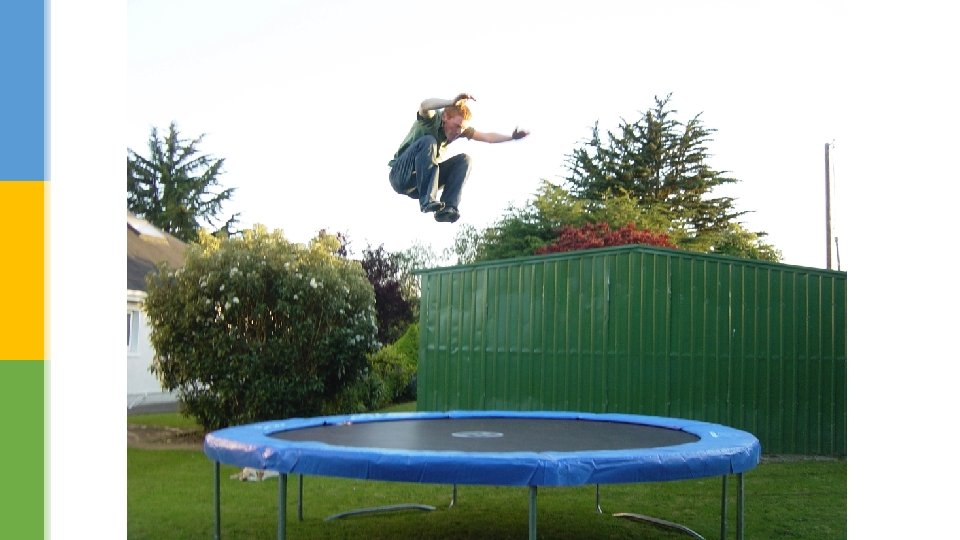Did you know…? § Engineers build in a safety margin when designing structures. § This reduces the chance of a structure failing if an unexpected force is applied or if materials gradually become weaker as they get older.Thank you